Function Repository Resource:

# SymmetricKroneckerProduct

Calculate the symmetric Kronecker product of two matrices

Contributed by: Jan Mangaldan
 ResourceFunction["SymmetricKroneckerProduct"][m1,m2] constructs the symmetric Kronecker product of the square matrices m1 and m2.

## Details

The symmetric Kronecker product is a variation of the Kronecker product that is commutative, and is often used in the theory of semidefinite programming, and algorithms for solving semidefinite programs.
The square matrices m1 and m2 must have the same dimensions.
If m1 and m2 are both of length n, then the symmetric Kronecker product is a square matrix of length n(n+1)/2.
ResourceFunction["SymmetricKroneckerProduct"] can be used on SparseArray objects, returning a SparseArray object when possible.

## Examples

### Basic Examples (1)

Compute the symmetric Kronecker product of two symbolic 2×2 matrices:

 In:=Out=### Scope (3)

The symmetric Kronecker product of two exact matrices:

 In:=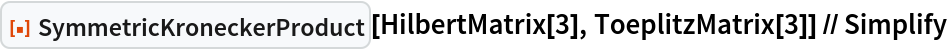Out=The symmetric Kronecker product of two numerical matrices:

 In:=Out=The symmetric Kronecker product of two sparse matrices:

 In:=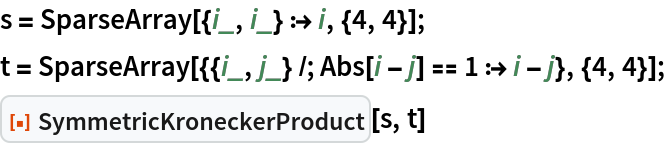Out=### Properties and Relations (5)

The symmetric Kronecker product is multi-linear (linear in each argument):In:=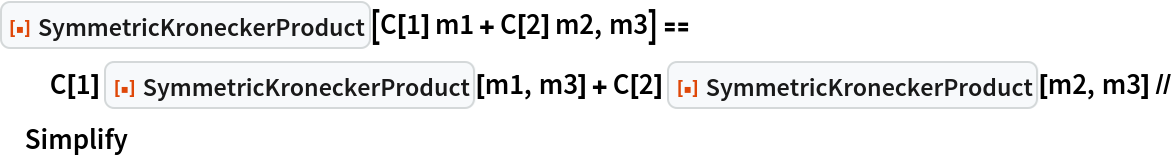Out=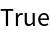In:=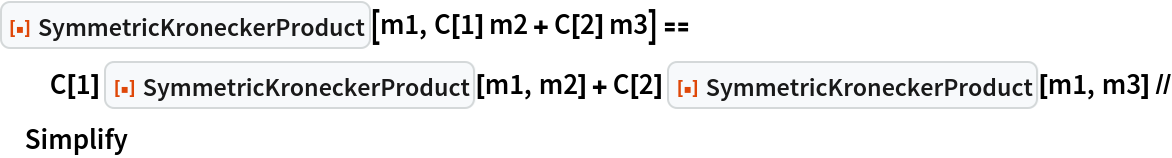Out=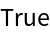The symmetric Kronecker product is commutative:

 In:=Out=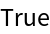Transposition distributes over the symmetric Kronecker product:

 In:=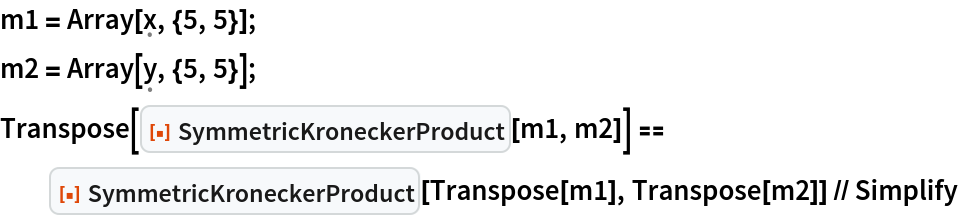Out=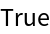The symmetric Kronecker product distributes over addition:In:=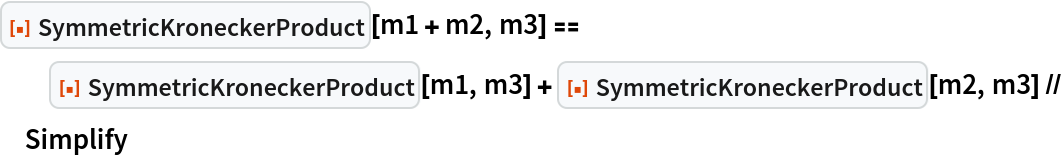Out=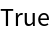Verify an expansion formula for the product of two symmetric Kronecker products:

 In:=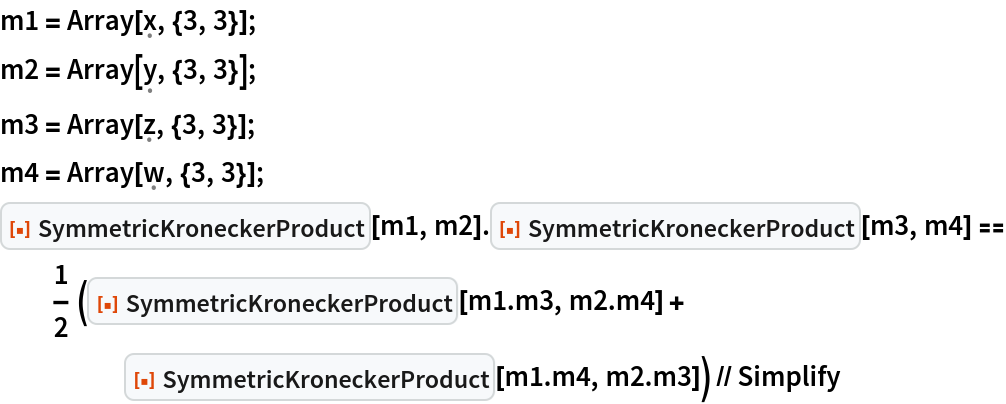Out=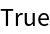## Version History

• 1.0.0 – 12 September 2022